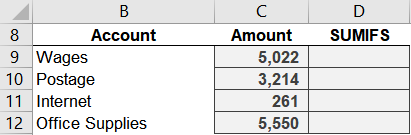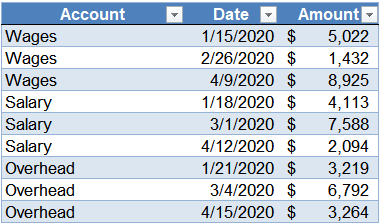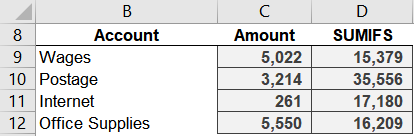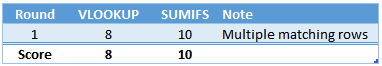# Battle of Heavyweights: Round 1It is time! In the first post, we met VLOOKUP. In the second post, we met SUMIFS. And now, the battle begins! Here is how it will work. The competition will consist of several rounds. In each round, we will use VLOOKUP and then SUMIFS to perform the same task. Then, we’ll determine who wins the round by deciding which function we would use in practice to accomplish the task.

The purpose of this format is to make it easy to compare and understand their key differences so that we are comfortable determining which to use in a given situation. Sound good?

Round 1 … begins … now!

## Round 1

In this round, we are trying to retrieve values for selected accounts from a data table. So, we begin by entering the desired accounts into the worksheet. We leave an empty column for the amount (which we’ll retrieve from our data table using a formula).But, since this is a battle, we’ll actually use two amount columns. One column for the results returned by VLOOKUP and the other column for the results of SUMIFS. Here is our updated worksheet:This will help us easily compare the results of our two heavyweights.

## VLOOKUP

Now, let’s use VLOOKUP to retrieve values from the data table, named Table1. We write the following formula into cell C9:

`=VLOOKUP(B9, Table1, 3, 0)`

We hit Enter and fill the formula down:No errors … good. We see some values … good.

VLOOKUP returned 5,022 for Wages. Let’s compare that value with our data table. We examine the data table and … hmmm:The first Wages row is indeed 5,022, however, there are multiple Wages rows. So, it appears as if VLOOKUP return the first one … and then stops.

And that is exactly right … VLOOKUP stops at the first matching row.

## SUMIFS

Let’s try this again, but this time with SUMIFS. We write the following formula into D9:

`=SUMIFS(Table1[Amount], Table1[Account], B9)`

We fill it down, and bam:Interesting … it appears as if SUMIFS returns the sum of all matching rows.

And that is exactly right. It looks through all rows in the range, and returns the sum of those that meet the condition (or conditions).

## Analysis

So, I want to call out our first key difference (and the point of this round):

VLOOKUP stops at the first matching row;

SUMIFS returns the sum of all matching rows

VLOOKUP and SUMIFS are different functions, designed for different purposes. And this is one of those key differences … VLOOKUP is designed to stop at the first match whereas SUMIFS is designed to return the sum of all matching rows.

Key point: when deciding which function to use in your workbook, you’ll want to determine if there are multiple matching rows in your data table.

As the rounds proceed, we’ll build a quick-reference scoring table to make it easier to recall the key differences and which function we prefer to use depending on the workbook, data, and goal. Since I’m presenting this series using a boxing metaphor, we may as well keep score like a normal boxing match.

We’ll use the standard 10-Point system, where the “winner” of each round is awarded 10 points. When we see this, we’ll remember which function we prefer for that specific task. The other function will score 8 or 9 points. It will receive 9 points if it is able to accomplish the task with the help of a friend (like a nested helper function), otherwise it will score 8. This way, if we see a 9 we’ll remember that the function can accomplish this task with a bit of assistance.

So … in Round 1, we award 10 points to SUMIFS and 8 points to VLOOKUP. That way, we can easily recall that when there are multiple matching rows, we prefer to use SUMIFS.We are just getting started and there are plenty of rounds to go!

See you in the next round 🙂

Sample file: Round1.xlsx

Get a quick email notice when a new Excel article is available

• This field is for validation purposes and should be left unchanged.